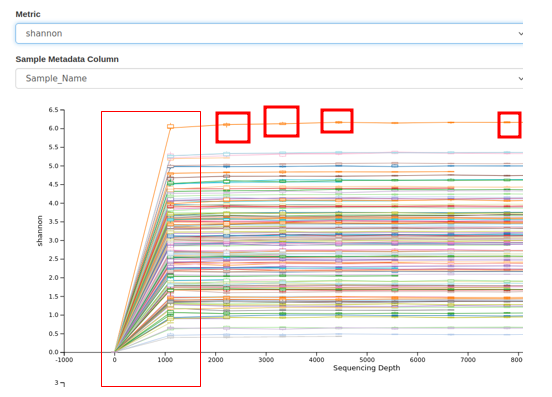# What is the bar graph of the rarefaction curves ? (Does it have variance ?)Hello everyone. I am trying to understand rarefaction curves.

As far as I understand, a rarefaction curve is a plot of the number of species (y-axis) against the number of read lengths (x-axis) in a sample. I know it has been used to estimate the diversity of a community by showing how the number of species increases as the read lengths of sample increases.

I couldn't understand the presence of the bar graph on the rarefaction curve. I think it shows the variance of the values. If one curve represents one diversity value of its own, can it have different diversity values when the same test is conducted repeatedly? (If one curve means one sample's number of species value.)

If my understanding is wrong, please teach me the concept of rarefaction more exactly and explain why the bar graph exists.

The x-axis is actually read depth--the number of reads chosen from a sample--not read length. That's why you see some lines stop short before the right margin of the figure--such samples don't have enough reads for the higher number samplings. At each simulated sequencing depth (read depth) multiple iterations of random sampling are performed (this is what the `--p-iterations` flag does). Thus, at each point you have a distribution of diversity values of n=iterations. It looks like these are being plotted with a box-plot (not a bar graph).